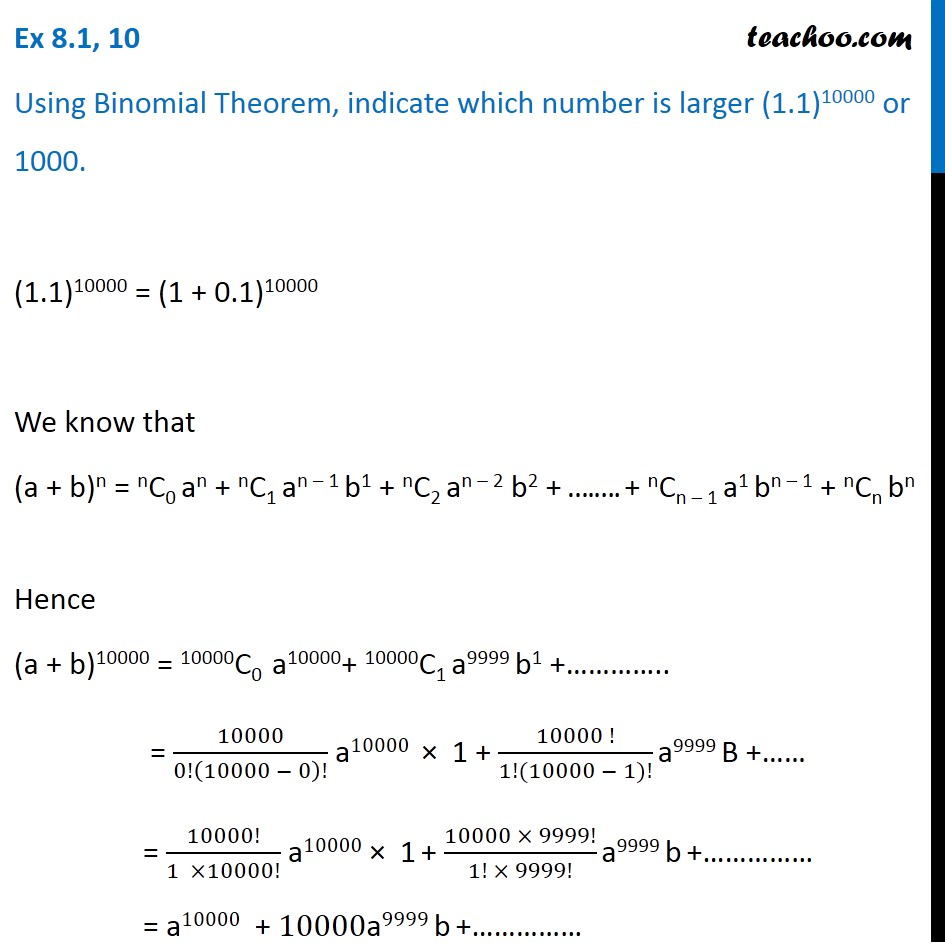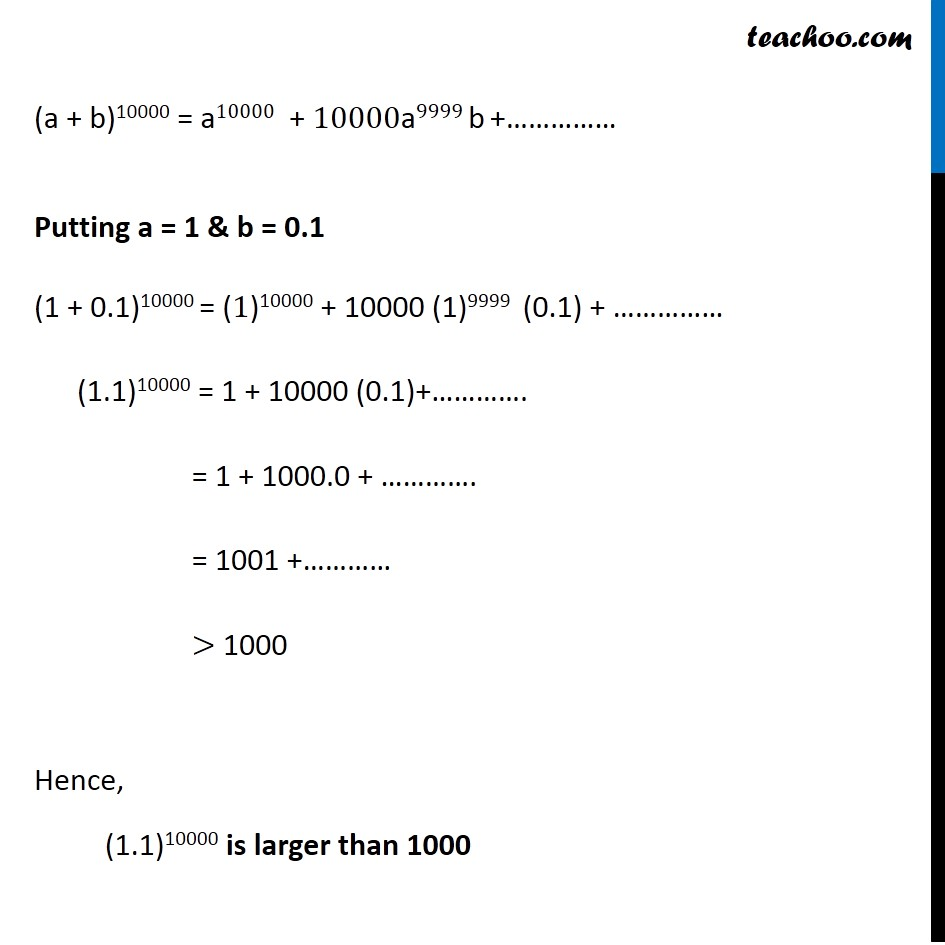Which is greater

Chapter 7 Class 11 Binomial Theorem
Concept wiseLearn in your speed, with individual attention - Teachoo Maths 1-on-1 Class

### Transcript

Ex 7.1, 10 Using Binomial Theorem, indicate which number is larger (1.1)10000 or 1000. (1.1)10000 = (1 + 0.1)10000 We know that (a + b)n = nC0 an + nC1 an – 1 b1 + nC2 an – 2 b2 + ….…. + nCn – 1 a1 bn – 1 + nCn bn Hence (a + b)10000 = 10000C0 a10000+ 10000C1 a9999 b1 +………….. = 10000/0!(10000 − 0)! a10000 × 1 + (10000 !)/(1!(10000 − 1)!) a9999 B +…… = 10000!/(1 ×10000!) a10000 × 1 + (10000 × 9999!)/(1! × 9999!) a9999 b +…………… = a10000 + 10000a9999 b +…………… (a + b)10000 = a10000 + 10000a9999 b +…………… Putting a = 1 & b = 0.1 (1 + 0.1)10000 = (1)10000 + 10000 (1)9999 (0.1) + …………… (1.1)10000 = 1 + 10000 (0.1)+…………. = 1 + 1000.0 + …………. = 1001 +………… > 1000 Hence, (1.1)10000 is larger than 1000